Language: Deutsch English

Last Update: 2022 - 04 - 15# Compute the quarter of a date in a calculated field in an Access table

by Philipp Stiefel, originally published February 21st, 2017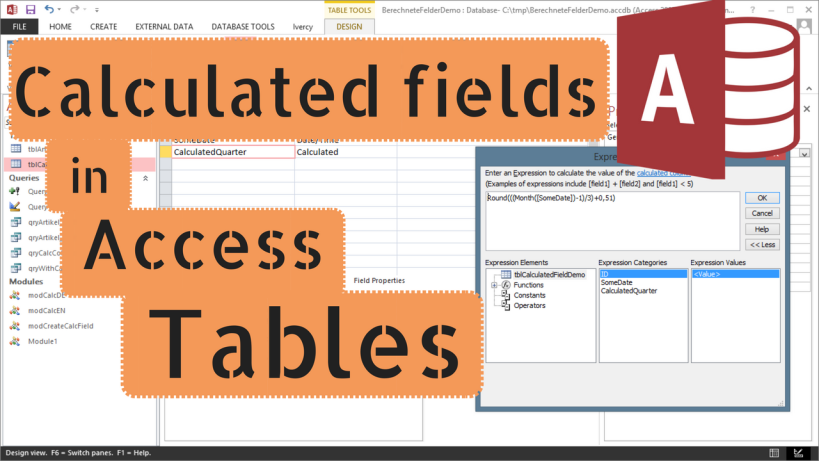Related Article: Calculated fields in Queries

Recently someone in an Access forum asked how to calculate the quarter of a date in a calculated table column. Only then I became aware that since Microsoft Access 2010 there is the possibility to create calculated fields in an Access Table.

In this article, I’m going to show you how to create calculated columns in tables in general as well as how to calculate the quarter of a date in such a calculated column.

## Creating a calculated field

To create a calculated field in a table, just follow these steps.

1. Create a new table or open an existing one in Design View.

If you created a new table save it before proceeding. Otherwise, the table’s columns will not show up in Expression Builder later.

2. Enter a name for your calculated field in the first empty row of the column names.

3. Select the data type Calculated for this new column

Access should now open the Expression Builder automatically. The next steps are only required if you closed the Expression Builder before you completed entering the expression.

4. In the Field Properties, on tab General find the line Expression. Click on the button with the three dots.

5. Use the Expression Builder dialog to create the expression required for your calculation.

6. It does not make a difference for the actual computation, still I would to recommend to select the proper Result Type for your expression.

### Steps 2 - 5 in pictures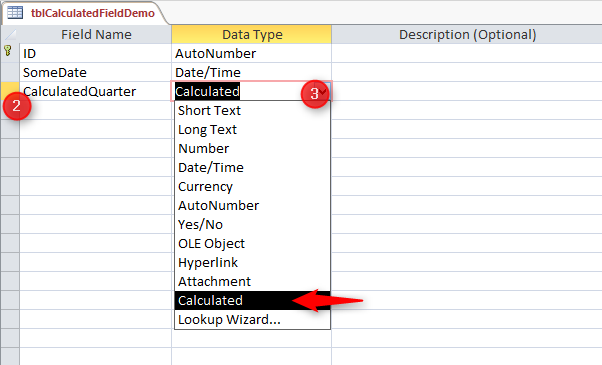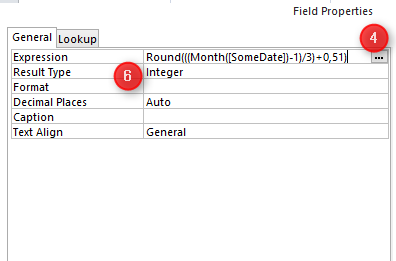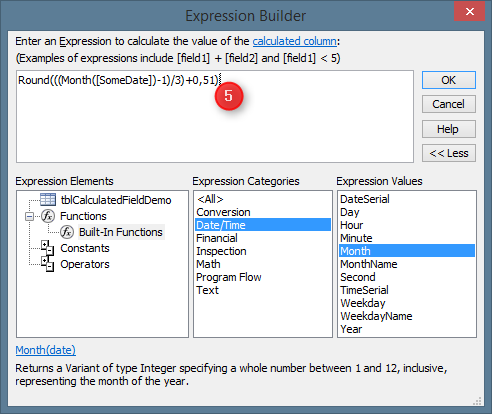Instead of opening and using the Expression Builder in step 4 and 5, you can of course just type your expression into the Expression field property directly. - Read the next paragraph first, though.

## Limited availability of functions

If you create a calculated field in a query, you can use any function built-into Access, available in the VBA Library, or your own, custom VBA-Function. However, when creating a calculated column in a table, the available functions are very limited.

So, even if you do not like the Expression Builder dialog in general and would rather prefer to write your calculation manually, it actually makes sense to use it in this specific context. If you invoke the Expression Builder from the properties window of a calculated field in a table, it will only show functions that you can actually use in this context.

If you can’t find a function within Expression Builder’s function list it is not available to be used for a calculated column in a table.

A general limitation of calculated table fields is that you can use only columns from the same table in your expression.

## Calculate the quarter of a date

In the actual forum question, which inspired me to write this article, someone wanted to calculate the quarter of a date in a calculated column. Usually, this is straightforward and can easily accomplished in an Access query using this expression:

Format([yourDateColumn];"q")

Not so much with a calculated field in a table. The Format-Function is strictly not allowed there.

There is a slightly more complicated way to calculate the quarter of a date based on mathematical calculation.

• Use the Month-Function to get the month,
• subtract one,
• divide by 3 and
• then “round up” to the next higher integer value.

Here is an expression that does the calculation described above:

Round(((Month([yourDateColumn])-1)/3)+0.51)

The “round up” above is in quotes because it does not strictly comply with the definition of rounding. We convert any number to the next higher integer even if the number has no decimal digits. The purpose of the +0.51 is to force the built-in Access/VBA Round-Function to always round up in the desired fashion.

This approach solves the problem of calculating the quarter of the date.

## Create calculated fields with code

To my knowledge, it is not possible to create a calculated table field using SQL. Regardless whether I executed an ALTER TABLE statement to add such a column via DAO or ADO, every attempt to do so resulted in a “Syntax error in field definition” message.

It is, however, possible to create a calculated field using VBA and the DAO.TableDef-Object. This is absolutely straightforward and does not differ much from creating any other column by code. You only need to set the Expression property of the created DAO.Field-Object to the expression performing your calculation.

Here is some sample code analogous to the manual operation above.

Public Sub CreateCalculatedQuarterField() Dim db As DAO.Database Dim td As DAO.TableDef Dim fld As DAO.Field2 Set db = CurrentDb() Set td = db.TableDefs("tblCalculatedFieldDemo") Set fld = td.CreateField("CalculatedQuarterWithVBA", dbInteger) fld.Expression = "Round(((Month([SomeDate])-1)/3)+0.51)" td.Fields.Append fld End Sub

It seems not to be possible to change the definition of an existing calculated field via VBA code, once the field was append to the Fields collection of the TableDef object. As the data in that column is calculated and so by definition is redundant, a very simple workaround is to simply delete the existing field by code and create a new field based on the new expression.

## Performance of calculated fields

It is very hard to find a definite statement on this in the Access documentation, but according to my own research Access does actually store the results of the calculations used in tables, instead of calculating them each time the result is required.

Unfortunately, other than with Microsoft SQL Server, it is not possible to index calculated fields in Access tables (tested with Acc2010 and Acc2013). So there is only a very small performance advantage to gain from putting calculations into tables instead of queries.

To give you an idea of the effects, I did a very rough performance comparison between a calculated column in a table and the same calculation in a query.

 Calculation in Query Calculation in Table Calculated Column Expression - Second([SomeDate]) Query criteria Second([SomeDate]) = 30 CalculatedTableColumn = 30 Total Rows 2,000,000 (2 Million) 2,000,000 (2 Million) Matching rows 1 1 Average execution time (Sample size: 100 executions) 2.1 seconds 1.25 seconds

For completeness sake, here is a chart comparing results: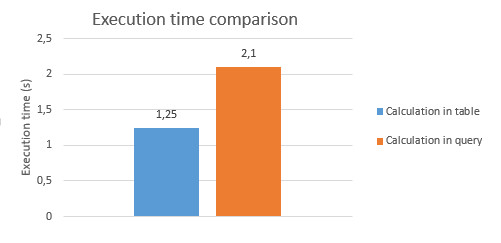This difference looks huge at first glance, but please be aware that this performance test is pretty construed.

• 2 million rows in an Access tables is quite a lot. I have seen tables that big in the real world, but they are fairly rare.
• Using a calculation based on table data in the criteria of a query should be avoided whenever possible. If your application depends heavily on filtering large volumes of data by calculated fields as the main criteria, this indicates a flaw in your database design.

In sensible scenarios there should be hardly any difference noticeable between a calculation in a query and one in a table.

So, the bottom line is, there might be a tiny performance benefit resulting from calculated table fields, but if this has a real significance in your application, there most likely are other options for optimisation, yielding far better results.

Issues with calculated fields

Please be aware that calculated columns were a new feature of Access 2010. A table containing a calculated field cannot be opened in previous versions of Microsoft Access.

There are texts on the internet, claiming that the stored data might not be recomputed when changing the underlying expression of a calculated column. - I tried to reproduce this issue, but, with Access 2010 + 2013 updated to the current patch level, I was unable to identify any situation where that actually happened.

## When to use calculated fields in tables

Please, always keep in mind that, in contrast to Excel spreadsheets, tables in relational databases are not meant for calculations. The primary purpose of relational tables is to store data.

In my opinion, it is a good practice to separate the data storage from business logic and application logic. If you use tables just to store data and put all calculations in higher layers of your application, like queries, this separation of plain data and calculated results is much clearer.

There are however two scenario where it might make sense to use a calculated field in a table.

• The calculated field is of central importance to the data in the table and you can safely assume that this field will be required almost everywhere where data from that table is used.
• You create an “open application” where users are allowed or even encouraged to create their own queries based on the data in the application. In this it can be an advantage to put basic calculations right into the tables. Then users do not need to be educated on the difference of the tables containing the plain data and queries retrieving the data and performing calculations.

Nevertheless, even in those cases I myself would prefer the classic approach of plain data storage in tables and calculations in queries only. This will require a bit of additional work in the above-mentioned scenarios, but I will happily put in the extra effort to achieve this separation of concerns.

## Companion Video

Here is a companion video I recorded for this article.

If you like the video, please give it a thumbs up on YouTube. - Thank you!